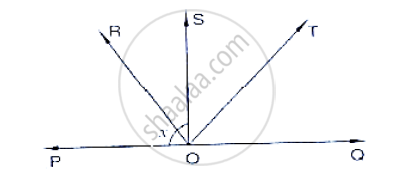# In Below Fig, Ray Os Stand on a Line Poq. Ray Or and Ray Ot Are Angle Bisectors of ∠Pos and ∠Soq Respectively. If ∠Pos = X, Find ∠Rot. - Mathematics

In below fig, ray OS stand on a line POQ. Ray OR and ray OT are angle bisectors of ∠POS
and ∠SOQ respectively. If ∠POS = x, find ∠ROT.#### Solution

Given,

Ray OS stand on a line POQ

Ray OR and Ray OT are angle bisectors of ÐPOS and ÐSOQ respectively

∠POS = x

∠POS and ∠QOS is linear pair

ÐPOS + ÐQOS = 180°

x + ÐQOS = 180°

∠QOS = 180 - x

Now, ray or bisector ∠POS

∴ ∠ROS = 1/2 ∠POS

= 1/2×  x                        [ ∵ ∠POS = x]

∠ROS = x

Similarly ray OT bisector ∠QOS

∵∠TOS = 1/2 ∠QOS

= 180/2 - x                  [∵  ∠QOS = 180 - x]

= 90/2 - x

∴ ∠ROT = ∠ROS + ∠ROT

= x/2+ 90 -x/2

= 90°

∴∠ROT = 90°

Concept: Pairs of Angles
Is there an error in this question or solution?
Chapter 10: Lines and Angles - Exercise 10.2 [Page 16]

#### APPEARS IN

RD Sharma Mathematics for Class 9
Chapter 10 Lines and Angles
Exercise 10.2 | Q 15 | Page 16

Share